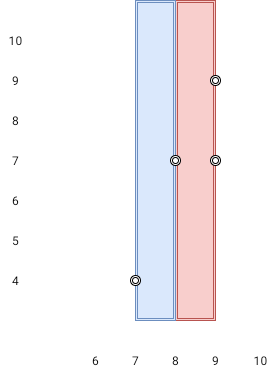# GeetCode Hub

Given `n` `points` on a 2D plane where `points[i] = [xi, yi]`, Return the widest vertical area between two points such that no points are inside the area.

A vertical area is an area of fixed-width extending infinitely along the y-axis (i.e., infinite height). The widest vertical area is the one with the maximum width.

Note that points on the edge of a vertical area are not considered included in the area.

Example 1:```Input: points = [[8,7],[9,9],[7,4],[9,7]]
Output: 1
Explanation: Both the red and the blue area are optimal.
```

Example 2:

```Input: points = [[3,1],[9,0],[1,0],[1,4],[5,3],[8,8]]
Output: 3
```

Constraints:

• `n == points.length`
• `2 <= n <= 105`
• `points[i].length == 2`
• `0 <= xi, yi <= 109`

class Solution { public int maxWidthOfVerticalArea(int[][] points) { } }